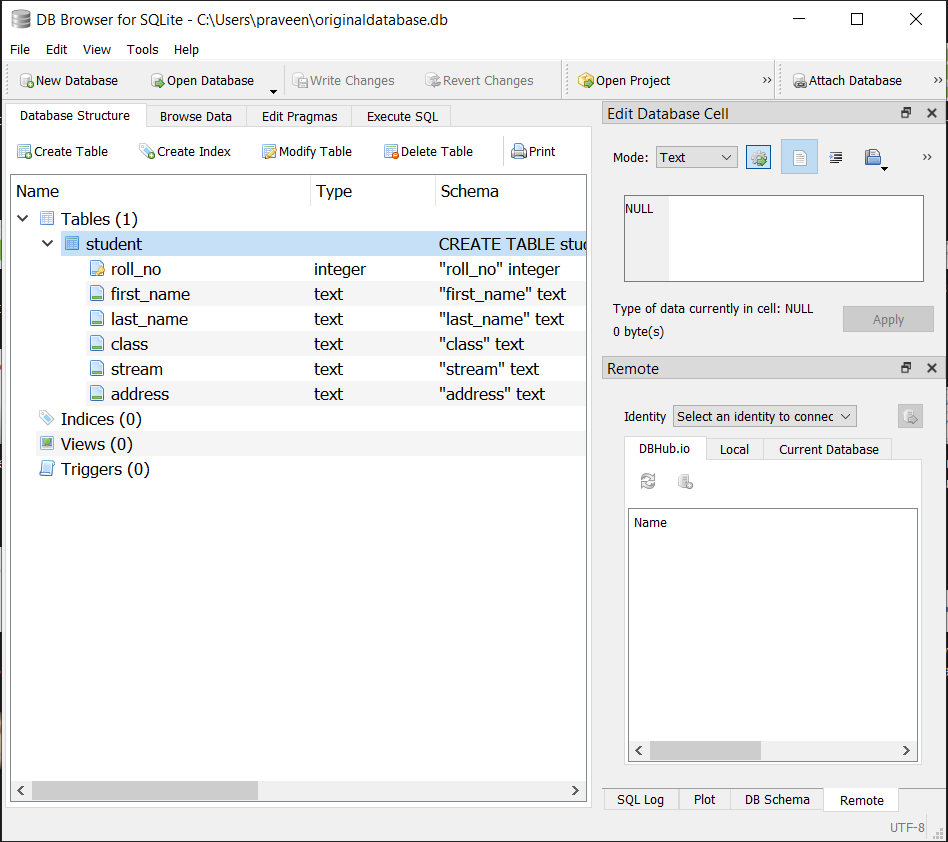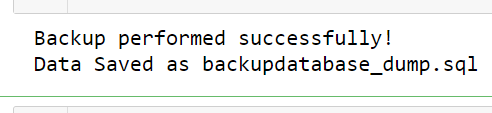Open in App
Not now

# How to Create a Backup of a SQLite Database using Python?

• Last Updated : 16 May, 2021

In this article, we will learn How to Create a Backup of an SQLite Database using Python. To Create a Backup of an SQLite Database using Python, the required modules are SQLite3 and IO.

First, let’s create the original database to do that follow the below program:

## Python3

 `import` `sqlite3``import` `io``from` `sqlite3 ``import` `Error`` ` ` ` `def` `SQLite_connection():``   ` `    ``try``:    ``        ``conn ``=` `sqlite3.connect(``'Originaldatabase.db'``)``        ``print``(``"Connection is established successfully!"``)``        ``print``(``"'originaldatabase.db' is created "``)``        ``return` `conn``       ` `    ``except` `Error:``        ``print``(Error)``         ` `    ``finally``:``        ``conn.close()`` ` `SQLite_connection()`

Output:Original database in memory:Then we will create a student Table in the original database. We will execute the SQLite syntax to create a table in the cursor object.

Syntax:

cursor_object = conn.cursor()

cursor_object.execute(“CREATE TABLE Table name()”)

Below is the complete program to create a student Table in the original database :

## Python3

 `import` `sqlite3``import` `io``from` `sqlite3 ``import` `Error`` ` ` ` `def` `sql_connection():``    ``try``:``        ``conn ``=` `sqlite3.connect(``'Originaldatabase.db'``)``        ``return` `conn``    ``except` `Error:``        ``print``(Error)`` ` ` ` `def` `sql_table(conn):``   ` `    ``cursor_object ``=` `conn.cursor()``    ``cursor_object.execute(``        ``"CREATE TABLE student(roll_no integer PRIMARY KEY,first_name text,\``        ``last_name text, ``class` `text, stream text,address text)")``    ``conn.commit()`` ` ` ` `conn ``=` `sql_connection()``sql_table(conn)`

To check if our table is created, we can use the DB Browser for SQLite to view our table. Open  ‘originaldatabase.db’ file with the program, and we should see our table:## Creating a backup of Database

We will create a backup of the database. To do that we will call the open() function from the IO module. This function will give the total number of database rows that will be modified, inserted, or deleted since the database connection was opened. Also, we will use iterdump() function. In an SQL text format iterdump() function gives an iterator to dump the database. Which is used to save an in-memory database for later restoration. In the sqlite3 shell, This function provides the same capabilities as the .dump command.

Syntax:

```with io.open('backupdatabase.sql', 'w') as p:
for line in conn.iterdump():
p.write('%s\n' % line)```

By given Syntax backup will be performed successfully and data will be Saved as backupdatabase_dump.sql.

## Python3

 `import` `sqlite3``import` `io``conn ``=` `sqlite3.connect(``'Originaldatabase.db'``)  `` ` `# Open() function ``with io.``open``(``'backupdatabase_dump.sql'``, ``'w'``) as p: ``         ` `    ``# iterdump() function``    ``for` `line ``in` `conn.iterdump(): ``         ` `        ``p.write(``'%s\n'` `%` `line)``     ` `print``(``' Backup performed successfully!'``)``print``(``' Data Saved as backupdatabase_dump.sql'``)`` ` `conn.close()`

Output:Back up of original database:My Personal Notes arrow_drop_up